## 用R模拟本次北京新冠疫情

• 张丹, 分析师/程序员/Quant: R,Java,Nodejs
• blog: http://blog.fens.me
• email: bsspirit@gmail.com1. 获取北京新冠疫情数据
2. R0基本传染数介绍
3. 用SIR模拟北京疫情，结果失真
4. 用SIR拟合算出R0，结果合理

## 1. 获取北京新冠疫情数据

``````# 设置工作路径
> setwd("C:/work/R/covid19")

# 如果没安装，请先安装nCov2019包
> #remotes::install_github("YuLab-SMU/nCov2019")

# 加载nCov2019包
> library(nCov2019)

# 下载最近数据
> x <- query()
Querying the latest data...
last update: 2022-07-05
Querying the global data...
Gloabl total  555324897  cases; and  6362663  deaths
Gloabl total affect country or areas: 230
Gloabl total recovered cases: 165929
last update: 2022-07-05
Querying the historical data...
Querying the vaccine data...
Total Candidates Programs : 51
Querying the therapeutics data...
Total Candidates Programs : 84
Query finish, each time you can launch query() to reflash the data
``````

``````> library(ggplot2)
> library(magrittr)
> library(reshape2)
>
> # 画图函数
> draw<-function(df){
+   dat<-melt(df,id.vars = c("date"))
+   g<-ggplot(data = dat, mapping = aes(x=date,y=value,colour=variable))
+   g<-g+geom_line() + geom_point()
+   g<-g+scale_y_log10()
+   g<-g+ggtitle("北京疫情统计")+xlab("日期")+ylab("Log(人数)")
+   g
+ }
``````

``````> hist<-x\$historical
> beijing<-hist["China","beijing"]

# 查看数据集
country province       date cases deaths recovered
6     China  beijing 2020-01-22    14      0         0
95    China  beijing 2020-01-23    22      0         0
184   China  beijing 2020-01-24    36      0         1
273   China  beijing 2020-01-25    41      0         2
362   China  beijing 2020-01-26    68      0         2
451   China  beijing 2020-01-27    80      1         2
``````

``````> beijing\$daily<-c(0,diff(beijing\$cases))

# 画图
> draw(beijing[,c("date", "cases" ,"deaths", "recovered","daily")])
``````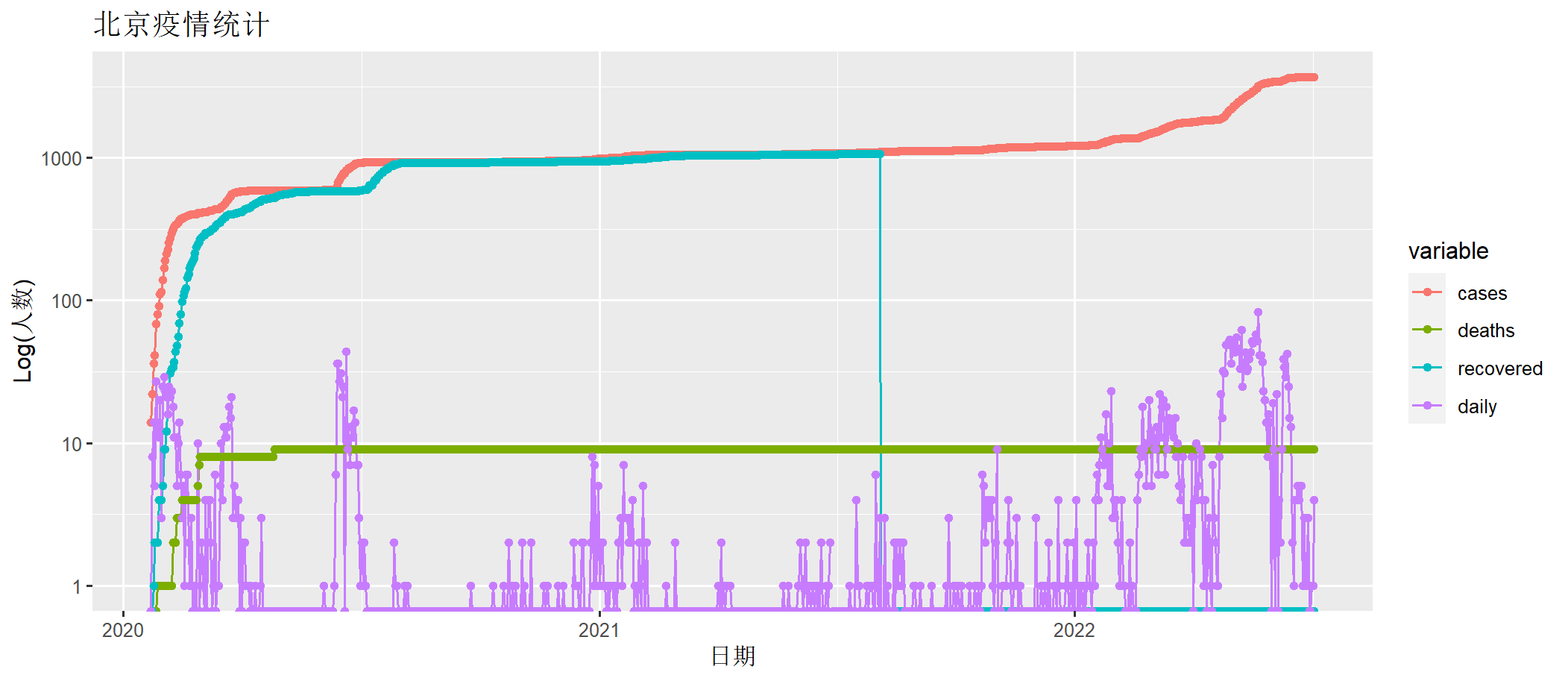``````# 取2022-04-01开始到2022-07-04数据
> bj202204<-beijing[which(beijing\$date>=as.Date("2022-04-01")),]
country province       date cases deaths recovered daily
71206   China  beijing 2022-04-01  1777      9         0     8
71295   China  beijing 2022-04-02  1777      9         0     0
71384   China  beijing 2022-04-03  1781      9         0     4
71473   China  beijing 2022-04-04  1791      9         0    10
71562   China  beijing 2022-04-05  1795      9         0     4
71651   China  beijing 2022-04-06  1800      9         0     5

# 计算这段时间的累计确诊数量
> bj202204\$cum<-cumsum(bj202204\$daily)

# 画图
> draw(bj202204[,c("date", "cum","daily")])
``````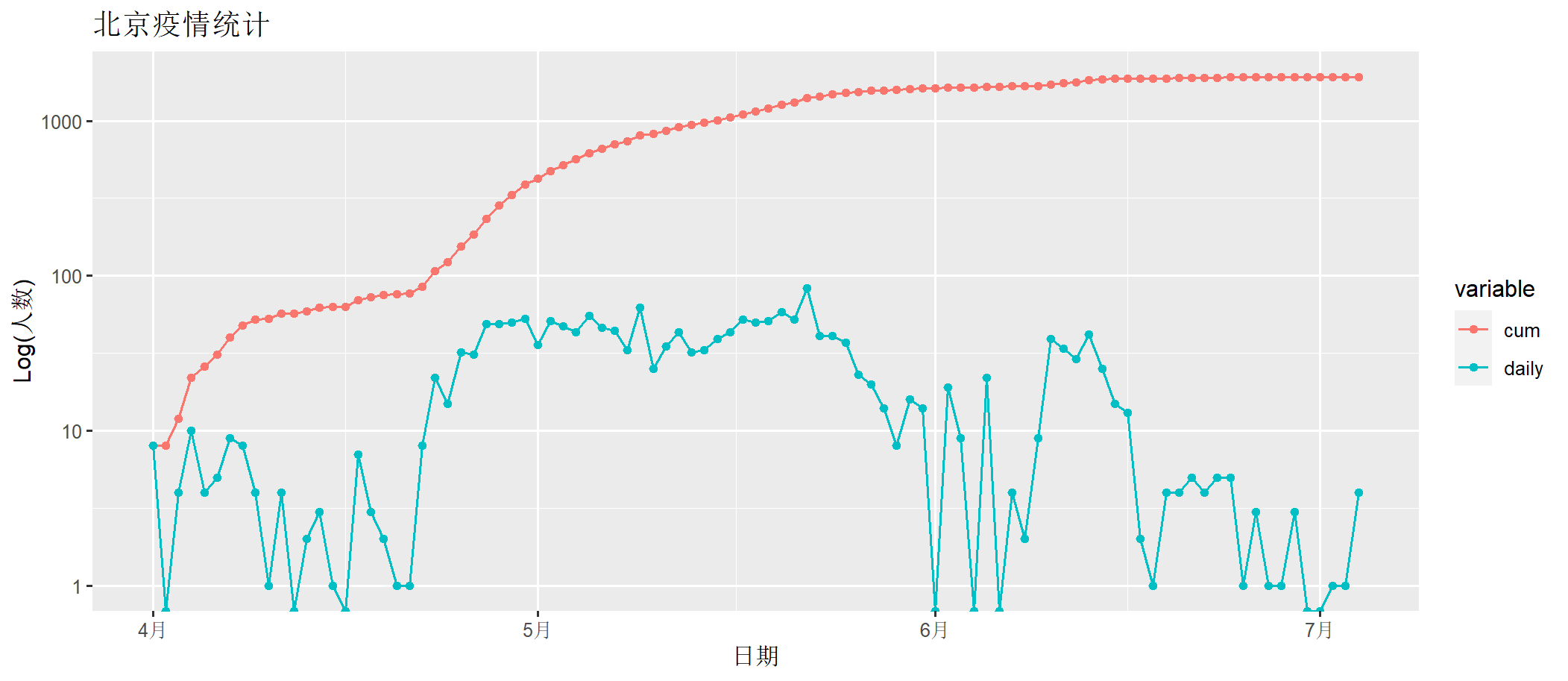## 2. R0基本传染数和Re有效传染数

2.1 R0基本传染数

R0基本传染数，这是一个很重要的传染病学概念，用来衡量病原体的传染性。R0是指在一个完全易感人群中（没有任何预防手段介入并且所有人对此病原体没有免疫力的情况下），一个病例能传染的平均人数。

R0最常用的方法是使用累积发病率数据。

``R0 = 传染期 * 每人每天的接触者数 * 每个接触者的感染概率``
• R0 < 1，意味着在没有防控的情况下， 一个感染者将传给少于一个人，所以传染病将会自行逐渐消失。
• R0 > 1，每个感染者能传染多于一个人，那么就会有发展成流行病（epidemic）的可能性。但是一般不会永远持续，因为可能被感染的人口会慢慢减少。部分人口可以死该传染病，部分则可能治愈后产生免疫力。
• R0 = 1，传染力维持，病院持续存在，会变成地方性流行病。

HIV/AIDS性传播4.5

SARS空气传播、 飞沫传播、粪口传播2-5

COVID-19飞沫传播、接触传播、粪口传播1.4-3.8
3.8–8.9（截至2020年6月）
Delta变异株：5.1
Alpha变异株：4–5
Omicron变异株：7

2.2 Re有效传染数

``````Re ＝ R0 *（1 – 有效控制率）*（易感人群比例）
``````
• 当Re>1时，每个受感染的个体将疾病传播给不止一个人，疾病可能在人群中传播。
• 当Re<1时，可能会发生个体病例传播给另一个人发生新的感染，要遏制疾病流行，Re必须小于1，疫苗通过减少易感人群的比例，是使Re < 1的最佳手段。

## 3. 用SIR模型模拟北京疫情

R程序实现代码

``````> library(EpiModel)
> param <- param.dcm(inf.prob = 0.5, act.rate = 0.5, rec.rate = 1/7)
> init <- init.dcm(s.num = 30*1000*1000, i.num = 8, r.num = 0)
> control <- control.dcm(type = "SIR", nsteps = 200, dt = 0.5)
> mod <- dcm(param, init, control)
``````

``````> mod
EpiModel Simulation
=======================
Model class: dcm

Simulation Summary
-----------------------
Model type: SIR
No. runs: 1
No. time steps: 200
No. groups: 1

Model Parameters
-----------------------
inf.prob = 0.5
act.rate = 0.5
rec.rate = 0.1428571

Model Output
-----------------------
Variables: s.num i.num r.num si.flow ir.flow num
``````

``````> plot(mod)
``````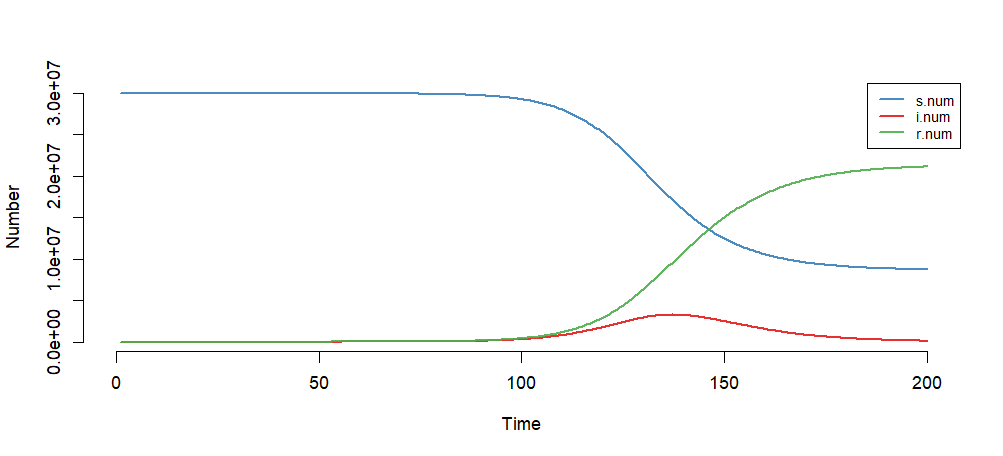``````
# 生成日期列
> moddf<-as.data.frame(mod)
> moddf\$date<-as.Date("2022-04-01")+0:(nrow(moddf)-1)

# 合并数据集
> d1<-data.frame(date=moddf\$date,i=moddf\$i.num,variable="sir")
> d2<-data.frame(date=bj202204\$date,i=bj202204\$cum,variable="real")
> mdf<-rbind(d1,d2)

# 画图
> g<-ggplot(data = mdf, mapping = aes(x=date,y=i,colour=variable))
> g<-g+geom_line() + geom_point()
> g<-g+scale_y_log10()
> g<-g+ggtitle("北京疫情统计")+xlab("日期")+ylab("Log(人数)")
> g
``````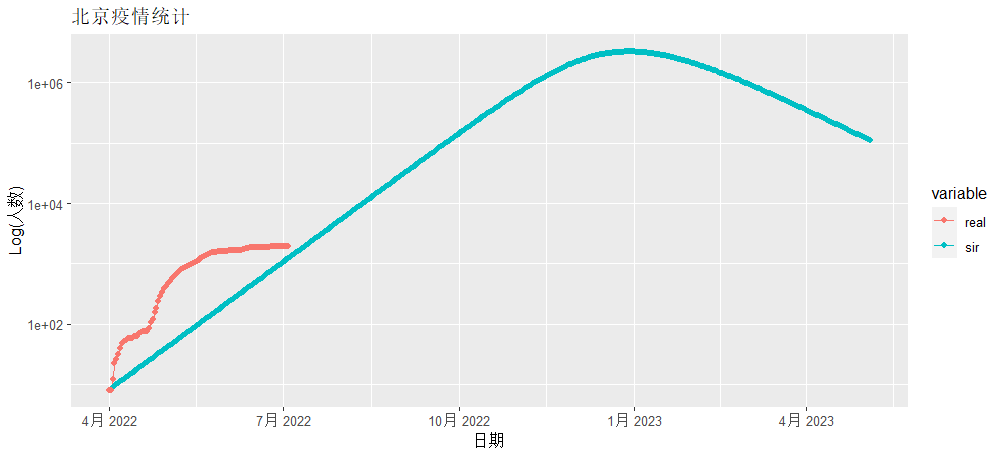## 4. 基于 SIR 拟合北京疫情

``````
# 加载程序包
> library(lubridate)
> library(plyr)

# 设置初始化参数
> cases <- bj202204\$cum     # 每日累计
> N <- 30*1000*1000         # 总人数
> startDay <- 6             # 开始累计时间

# 计算每日累计确诊
> infected <- cases[startDay:length(cases)]
> infected
   31   40   48   52   53   57   57   59   62   63   63   70   73   75   76   77   85  107  122  154
  185  234  283  333  386  422  473  520  563  618  664  708  741  803  828  863  906  938  971 1010
 1053 1105 1155 1206 1264 1316 1399 1440 1481 1518 1541 1561 1575 1583 1599 1613 1613 1632 1641 1641
 1663 1663 1667 1669 1678 1717 1751 1780 1822 1847 1862 1875 1877 1878 1882 1886 1891 1895 1900 1905
 1906 1909 1910 1911 1914 1914 1914 1915 1916 1920

# 以多少天为窗口计算R0
> window <- 12
``````

``````
# 定义SIR模型的拟合函数
> SIR<-function(time,vars,params){
+   with(as.list(c(vars,params)),{ #beta=rio,gamma=mu
+     num <- s.num + i.num + r.num
+     dS<- -beta * i.num * s.num/num
+     dI<- beta * i.num * s.num/num - gamma * i.num
+     dR<- gamma * i.num
+     return(list(c(dS,dI,dR)))
+   })
+ }
``````

``````
# 查看mod_SIR_1g_cl函数定义
> mod_SIR_1g_cl
function (t, t0, parms)
{
with(as.list(c(t0, parms)), {
num <- s.num + i.num + r.num
lambda <- inf.prob * act.rate * i.num/num
if (!is.null(parms\$inter.eff) && t >= inter.start) {
lambda <- lambda * (1 - inter.eff)
}
si.flow <- lambda * s.num
ir.flow <- rec.rate * i.num
dS <- -si.flow
dI <- si.flow - ir.flow
dR <- ir.flow
list(c(dS, dI, dR, si.flow, ir.flow), num = num)
})
}
``````

``````
> mse <- function(infected, fit) {
+   sum((infected - fit)^2) / length(infected)
+ }
> r2 <- function(infected, fit) {
+   1 - mse(infected, fit)/var(infected)
+ }
``````

``````> R0 <- function(days){
+
+   # 取窗口期内的数据拟合
+   WindowInfected <- infected[(days - window):(days - 1)]
+   # 持续天数
+   Days <- 1:(length(WindowInfected))
+
+   # 设置SIR模型的默认值，s.num易感人群，i.num已感人群，r.num已治愈人群
+   init <- c(s.num = N - WindowInfected, i.num = WindowInfected, r.num = 0)
+
+   # 定义损失函数
+   LOSS <- function(parameters) {
+     names(parameters) <- c("beta", "gamma")
+     out <- ode(y = init, times = Days, func = SIR, parms = parameters)
+     fit <- out[ , 3]
+     -r2(WindowInfected,fit)
+   }
+
+   # 拟合计算
+   Opt = optim(c(0.5, 0.5), LOSS, method = "L-BFGS-B", lower = c(0, 0), upper = c(Inf, 1))
+   Opt_par = setNames(Opt\$par, c("beta", "gamma"))
+   data.frame(days = days,
+              R0 = Opt_par["beta"] / Opt_par["gamma"],
+              beta = Opt_par["beta"],
+              gamma = Opt_par["gamma"],
+              value = Opt\$value,
+              date = ymd("2022-04-01") + days(startDay + days - 1) - 1) # StartDay - 1 | days - 1
+ }
``````

``````
> # 找到需要计算的日期
> days<-(window+1):(length(infected));days
 13 14 15 16 17 18 19 20 21 22 23 24 25 26 27 28 29 30 31 32 33 34 35 36 37 38 39 40 41 42 43 44 45 46
 47 48 49 50 51 52 53 54 55 56 57 58 59 60 61 62 63 64 65 66 67 68 69 70 71 72 73 74 75 76 77 78 79 80
 81 82 83 84 85 86 87 88 89 90

> # 计算R0的计算
> RODF<-lapply(days,R0) %>% ldply
days       R0      beta     gamma      value       date
1   13 1.163701 0.5813041 0.4995306 -0.5091804 2022-04-18
2   14 1.115407 0.5574453 0.4997684 -0.7966765 2022-04-19
3   15 1.081334 0.5405033 0.4998486 -0.9677076 2022-04-20
4   16 1.070063 0.5348910 0.4998687 -0.9729603 2022-04-21
5   17 1.072671 0.5362001 0.4998738 -0.9679795 2022-04-22
6   18 1.065505 0.5326116 0.4998677 -0.9529303 2022-04-23
``````

``````> plot(R0~date,data=RODF)
``````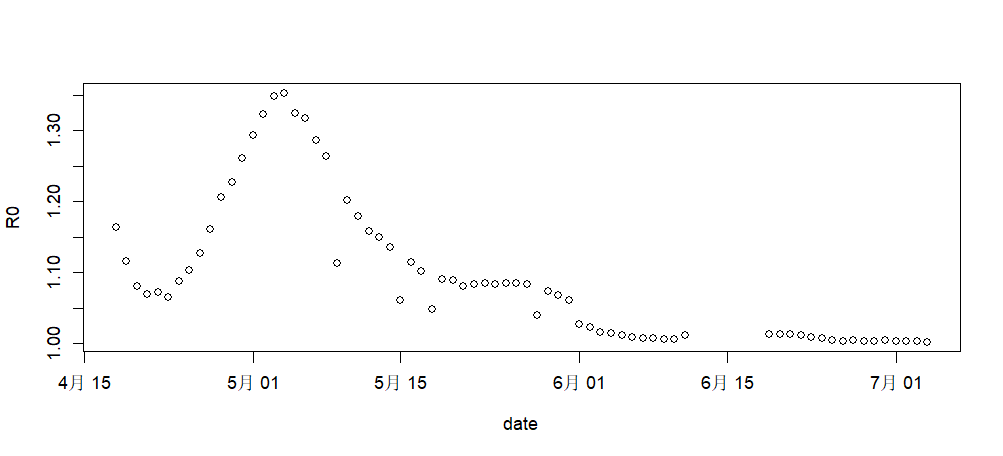``````> par(mfrow = c(3, 1))
> plot(cum~date,data=bj202204,type='b',col="blue")
> plot(daily~date,data=bj202204,type='b',col="red")
>
> d2<-data.frame(date=RODF\$date,R0=RODF\$R0)
> d1<-data.frame(date=as.Date('2022-04-01')+0:16,R0=NA)
> d2<-rbind(d1,d2)
> plot(R0~date,data=d2,type='b',col="orange")
``````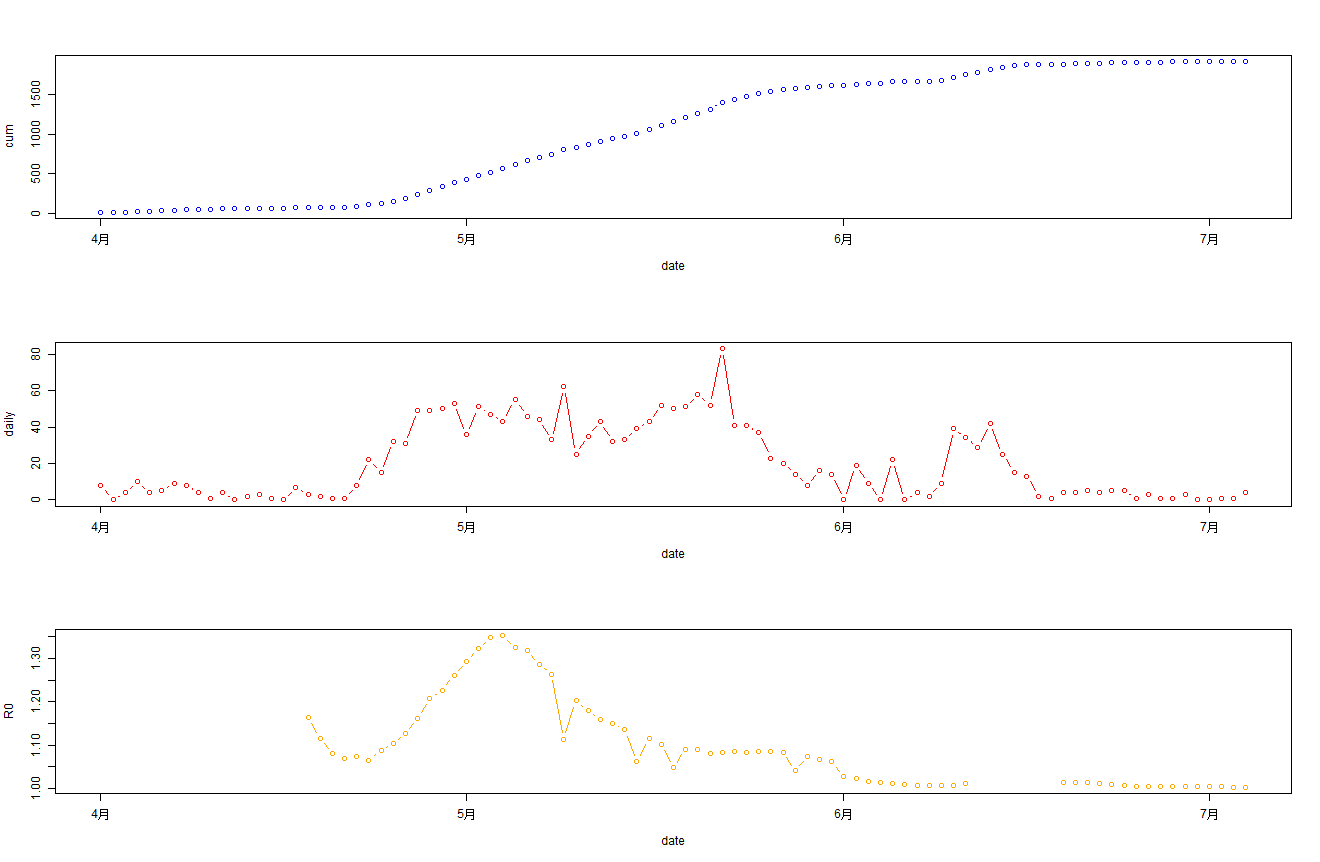This entry was posted in R语言实践

Article Rating
Subscribe
Notify ofThis site uses Akismet to reduce spam. Learn how your comment data is processed.

Inline Feedbacks